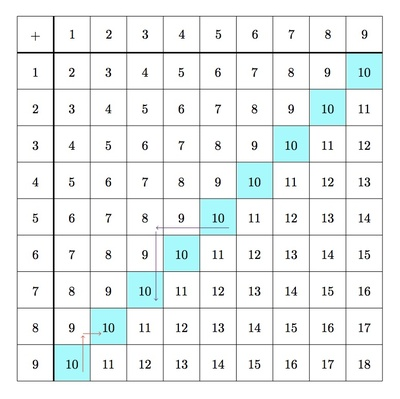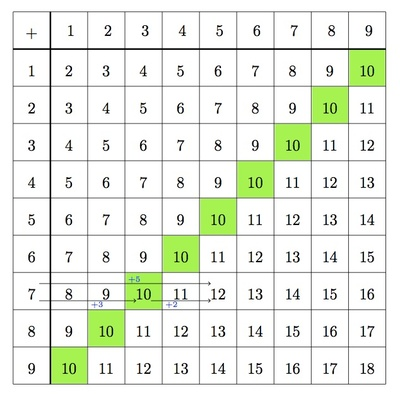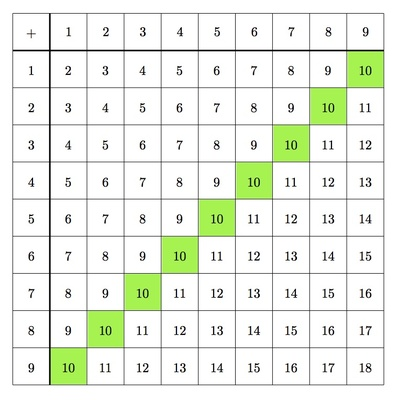# Making a ten

Alignments to Content Standards: 1.OA.C.6

#### Materials

• Copies of a table of sums for numbers 1 through 9:• Colored markers or crayons

#### Actions

1. Ask the students to shade in the 10's in the table. What do they notice about where these 10's are in the table?
2. Point out that if they start from the 10 in the bottom left corner and follow the red arrows shown below, they get to another 10:Ask them to explain what that path means and why they get to another 10.

3. Point out that if they start from the 10 in the 5+5 box and follow the purple arrows shown above, they get to another 10. Ask them to explain what that path means and why they get to another 10.

## IM Commentary

Making a 10 is an important technique to help students master single digit addition. This task is designed to help students visualize where the 10's are on a single digit addition table and explain why this is so. This knowledge can then be used to help them learn the addition table. For example, a way to find 7+5 visually using the addition table is shown below:Here 5 is broken up as 3+2 since 7 is 3 short of 10. In symbols, this can be written as

\begin{align} 7 + 5 &= 7 + 3 + 2 \\ &= 10 + 2 \\ &= 12 \end{align}

The task can help students with a strong geometric and visual intuition use these skills to help with their arithmetic. This task is intended for instructional purposes. Mastery of the ability of students to make a ten can be assessed by more direct means.

## Solution

1. Students will likely use many different coloring schemes. Here is an example where the 10's are all shaded in green:The 10's are on the diagonal going from the bottom left of the table to the top right.

2. The 10 in the bottom left is in the 9+1 box. Following the first red arrow moves us to the 8+1 box. This is 9, one less than 10. The second red arrow moves us to the 8+2 box. This is one more than 8+1=9 so it is also a 10.

Moving up one box subtracts one from 10 and moving to the right one box adds one to 9. After subtracting one and adding one we still have 10.

3. Two boxes to the left of 5+5 = 10 is 5+3 = 8. This is two less than 10. Moving down two boxes takes us to 7+3. This is two more than 5+3 = 8 so it is also a 10. <\p>

Moving two boxes to the left subtracts two from 10 and moving down two boxes adds two to 8. After subtracting two and adding two we will still have 10.